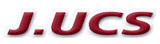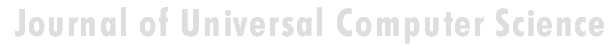Search
 Submission Procedure share: |available in:   PDF (120 kB) PS (129 kB)

get:
 Similar Docs BibTeX Write a comment
get:

DOI:   10.3217/jucs-011-12-2086

### A Direct Proof of the Equivalence between Brouwer's Fan Theorem and König's Lemma with a Uniqueness Hypothesis

Helmut Schwichtenberg (Mathematisches Institut, Universität München, Germany)

Abstract: From results of Ishihara it is known that the weak (that is, binary) form of König's lemma (WKL) implies Brouwer's fan theorem (Fan). Moreover, Berger and Ishihara [MLQ 2005] have shown that a weakened form WKL! of WKL, where as an additional hypothesis it is required that in an effective sense infinite paths are unique, is equivalent to Fan. The proof that WKL! implies Fan is done explicitely. The other direction (Fan implies WKL!) is far less directly proved; the emphasis is rather to provide a fair number of equivalents to Fan, and to do the proofs economically by giving a circle of implications. Here we give a direct construction. Moreover, we go one step further and formalize the equivalence proof (in the Minlog proof assistant). Since the statements of both Fan and WKL! have computational content, we can automatically extract terms from the two proofs. It turns out that these terms express in a rather perspicuous way the informal constructions.

Keywords: Brouwer's fan theorem, König's lemma

Categories: F.1